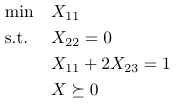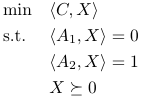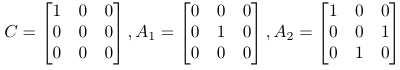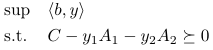# SDP and its dual in cvx

I am not sure if my code is correct although it can run without errors.

If I have the following SDP problem:I can rewrite the above as the following:whereAnd its dual problem is:The primal problem can be solved by cvx as following:

cvx_begin sdp
variable X(3,3) symmetric;
X == semidefinite(3)

minimize(C( : )’*X( : )); \ \ inner product of matrices

subject to
A1( : )’*X( : ) == 0;
A2( : )’*X( : ) == 1;
cvx_end

Now, I am not pretty much sure if the dual of it is correct:

cvx_begin sdp
variable X(3,3) symmetric;
dual variables y1 y2
X == semidefinite(3)

minimize(C( : )’*X( : ));

subject to
y1: A1( : )’*X( : ) == 0;
y2: A2( : )’*X( : ) == 1;
cvx_end

Since there are two equality constraints, I am not sure if the dual part in the code is correct.

You can combine the two equality constraints into one bigger equality constraint, and work with ttat if that fits within your comfort zone for taking duals.

When you solve both the primal and dual problems, you should get the same optimal objective value.

1 Like